# VBA CounterArticle byExcelMojo Team## What is Excel VBA Counter?

A VBA counter is a variable used to keep track of the number of iterations or occurrences within a loop or a specific task. It is commonly employed when you need to repeat a certain action a number of times or when you want to count the occurrences of a particular event or condition.

A VBA counter variable is typically declared and initialized before the loop or task begins. It is then incremented or modified within the loop or task to keep track of the count. Let us look at an example. In this example, we will create a basic loop that iterates from 1 to 10, utilizing a VBA counter variable to maintain a tally of the number of iterations. We can effectively determine the total count by incrementing the counter within the loop.

The output for the above VBA code will be a message box displaying the final value of the counter variable. In this case, since the loop iterates from 1 to 10 and increments the counter by 1 in each iteration, the counter will reach a final value of 10. So, the output message box will show: “The counter value is:10.”

###### Key Takeaways
• A VBA counter is a variable used to keep track of the number of iterations or occurrences within a loop or task in Excel VBA.
• To create a VBA counter, declare and initialize a counter variable, use a loop or code block to perform the desired actions, and increment or modify the counter variable within the loop.
• Examples of VBA counters include counting odd numbers, summing numbers, and tracking events based on specific conditions.
• When working with VBA counters,  correctly increment the counter, manage the scope of the counter variable, ensure proper initialization, select appropriate data types, and reset the counter if needed.

### How to Create an Excel VBA Counter?

To create an Excel VBA counter, follow these steps:

1. Open Excel and press ALT+F11 to open the Visual Basic Editor (VBE).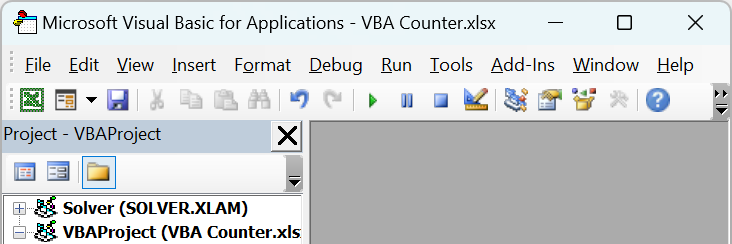2. Insert a new module by clicking “Insert” in the menu and selecting “Module.”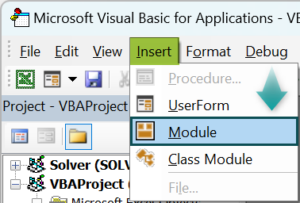3. In the module window, declare and initialize a counter variable. For example, let’s use a variable named “counter” and initialize it to zero: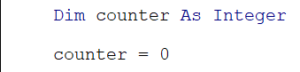4. Write a loop or code block where you want to use the counter. For example, let’s create a For loop that increments the counter from 1 to 10: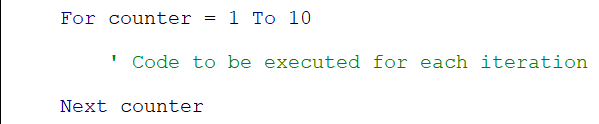5. Based on the counter value, you can perform actions or conditions within the loop. For example, you might want to display the current value of the counter: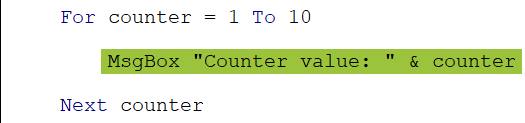6. Run the code by pressing F5 or by clicking the “Run” button in the VBE toolbar. The counter will increment with each iteration of the loop, and the desired actions or conditions can be performed accordingly.

Sub CounterExample()
Dim counter As Integer
counter = 0
For counter = 1 To 10
‘ Code to be executed for each iteration
MsgBox “Counter value: ” & counter
Next counter
End Sub

In this case, it will display the counter value from 1 to 10.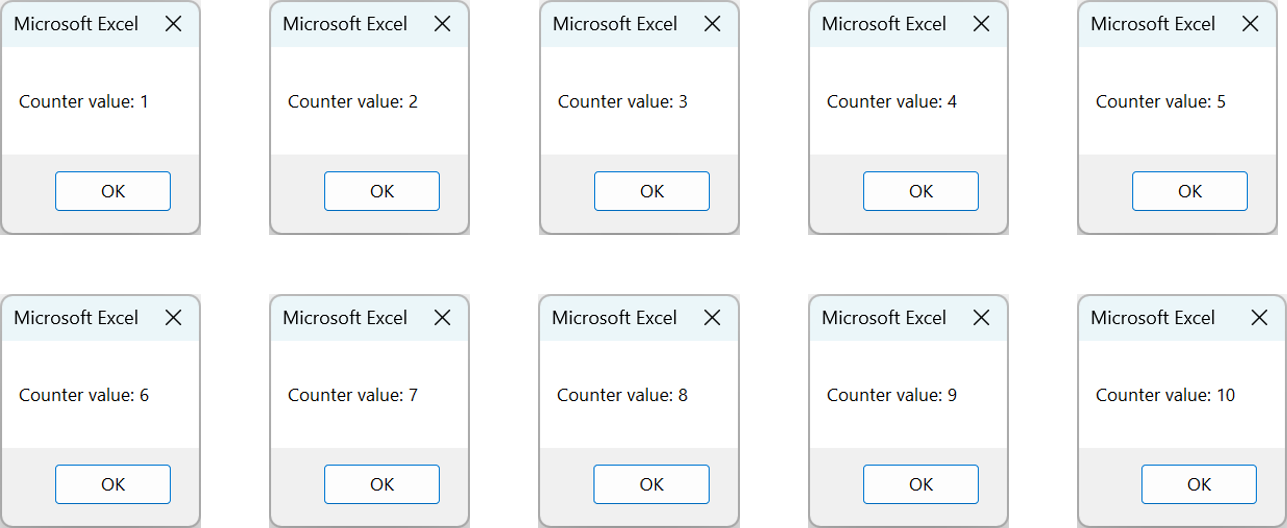### Examples

Let us look at some interesting examples using the VBA counter variable.

#### Example #1

In this example, the counter is used to count the number of odd numbers between 1 and 20. The code checks each number and increments the counter only if the number is odd.

• Step 1: In the new module, we begin by declaring the subroutine “CountOddNumbers” and declare two variables: “counter” and “num.” Both variables are of Integer data type. Additionally, we initialize the VBA counter variable with an initial value of 0.
• Step 2: We use a “For” loop structure to enter a loop. This loop allows us to iterate over numbers from 1 to 20. The “num” variable is the loop control variable, taking on each value within the specified range.
• Step 3: Inside the loop, we employ an “If” statement to check if the current value of “num” is an odd number. It is done by evaluating whether the number modulo 2 is not equal to 0. If the condition is true, it indicates that the number is odd.
• Step 4: Upon determining the odd number, we increment the counter variable by 1. This incrementation ensures that we keep track of the count of odd numbers encountered during the loop.
• Step 5: The loop continues to iterate until all the numbers from 1 to 20 have been processed. The “num” variable takes on the next value within the specified range in each iteration.
• Step 6: After the loop completes, we display the count of odd numbers encountered. It is achieved by utilizing a message box. The message box showcases the text “Number of odd numbers between 1 and 20: “, concatenated with the value of the counter variable.
• Step 7: Save the module and close the VBA. Now press Alt + F8 to open the Macro menu, select “CountOddNumbers” and run.
• Step 8: Once you execute the code, you will see the message box with the required output.

Here is the full code:

Sub CountOddNumbers()
Dim counter As Integer
Dim num As Integer
counter = 0
For num = 1 To 20
If num Mod 2 <> 0 Then
counter = counter + 1
End If
Next num
MsgBox “Number of odd numbers between 1 and 20: ” & counter
End Sub

#### Example #2

In this example, the VBA counter is employed to sum the first five numbers. The code uses a Do-While loop to iterate five times, accumulating the numbers in a sum variable.

• Step 1: In the new module, we begin by declaring the subroutine “SumNumbers” and two variables: “counter” and “sum.” Both variables are of Integer data type. These variables will be used to keep track of the count and the running sum, respectively.
• Step 2: Next, we initialize the counter variable with an initial value of 1 and the sum variable with an initial value of 0. This prepares the variables for the upcoming loop.
• Step 3: Next, we utilize a “Do While” loop to repeat actions until a specified condition is no longer met. In this case, the VBA loop will continue executing if the counter is less than or equal to 5.
• Step 4: Within the loop, we add the current value of the counter to the running sum. The statement “sum = sum + counter” ensures that the counter value is added to the existing sum.
• Step 5: Additionally, we increment the counter variable by 1 in each iteration using the statement “counter = counter + 1”. This allows us to move forward and consider the next number in the sequence.

The loop continues until the condition specified in the “Do While” statement is no longer true, indicating that the counter has reached a value greater than 5.

• Step 6: After the loop concludes, we display the final sum of the first five numbers encountered. It is accomplished by employing a message box that shows the text “Sum of the first five numbers:” followed by the value of the sum variable.
• Step 7: Save the module and close the VBA. Now press Alt + F8 to open the Macro menu, select “SumNumbers” and run.
• Step 8: Once you execute the code, you will see the message box with the required output.

Here is the full code:

Sub SumNumbers()
Dim counter As Integer
Dim sum As Integer
counter = 1
sum = 0
Do While counter <= 5
sum = sum + counter
counter = counter + 1
Loop
MsgBox “Sum of the first five numbers: ” & sum
End Sub

#### Example #3

In this scenario, the VBA counter is used to count the number of times a specific event occurs.

• Step 1: We begin by declaring the subroutine “TrackEvent” and two variables: “counter” and “eventOccurred.”

The “counter” variable is of Integer data type and will keep track of the count of event occurrences. The “eventOccurred” variable is a Boolean type, which will be used to track whether an event has occurred.

• Step 2: Next, we initialize the counter variable with an initial value of 0 to ensure a starting count of zero. Additionally, we set the eventOccurred variable to False to indicate that no event has occurred yet.
• Step 3: We enter a “Do While” loop in this step. This loop will continue executing as long as the counter is less than 5, meaning we want to track the event’s occurrence five times.
• Step 4: Within the loop, we simulate an event occurrence. For demonstration purposes, we generate a random number between 1 and 10 using the “Rnd” and “Int” functions. This random number simulates the occurrence of an event.
• Step 5: Using the Mod operator, we then check if the random number generated is divisible evenly by 2 (i.e., an even number). If the condition is true, it indicates that the event has occurred.
• Step 6: If the event has occurred, we set the eventOccurred variable to True and increment the counter variable by 1. It helps us keep track of the number of event occurrences.

The loop continues until the counter reaches a value of 5, indicating that the event has occurred five times.

• Step 7: After the loop concludes, we display the count of event occurrences. Using a message box, we show the text “Number of events occurred: ” concatenated with the value of the counter variable.
• Step 8: Save the module and close the VBA. Now press Alt + F8 to open the Macro menu and select “TrackEvent” and run.
• Step 9: Once you execute the code, the required output is displayed in the message box.

Here is the full code:

Sub TrackEvent()
Dim counter As Integer
Dim eventOccurred As Boolean
counter = 0
eventOccurred = False
Do While counter < 5
‘ Simulating an event occurrence
‘ For demonstration purposes, we’ll use a random number between 1 and 10
Dim randomNumber As Integer
randomNumber = Int((10 – 1 + 1) * Rnd + 1)
If randomNumber Mod 2 = 0 Then
eventOccurred = True
counter = counter + 1
End If
Loop
MsgBox “Number of events occurred: ” & counter
End Sub

### Important Things to Note

• Ensure the VBA counter variable is incremented or modified correctly within the loop or task to avoid infinite loops or incorrect counts. Failing to VBA counter increment may result in an endless loop.
• The scope of the VBA counter variable determines where it can be accessed and modified. If the VBA counter variable is declared within a specific procedure or VBA counter loop, it will only be accessible within that scope. If you need to access the counter from multiple procedures or loops, declare it at a higher scope (e.g., at the module level).
• When using a VBA counter time for time-based operations, consider the execution speed of your code and potential variations in system resources to ensure accurate time measurements.
• If you need to reset the counter during the execution of your code, simply assign the desired value to the counter variable. It can be useful when counting multiple occurrences or repeating a task with a different count.

1. Why is VBA Counter not working?

There can be several reasons why a VBA counter may not work. Common issues include not properly incrementing the counter within the loop, using an incorrect scope for the counter variable, or forgetting to initialize the counter. Carefully review your code to ensure the counter is incremented correctly and that all necessary conditions are met for proper counting.

2. What is the difference between a VBA Counter and VBA Count?

VBA counter is a variable used to keep track of the number of iterations or occurrences within a loop or task. It is a custom variable created by the programmer.
On the other hand, the VBA Count function is a built-in function used to count the number of elements in a collection or the occurrences of a specific value in an array. The Count function does not require manual declaration and initialization of a counter variable.

3. What is the difference between a VBA Counter and a For…Next loop?

A VBA counter is a variable that keeps track of the number of iterations or occurrences within a loop or task. It can be used in different types of loops, including For…Next loops.
A For…Next loop is a specific type of loop structure that automatically iterates a specified number of times. The counter variable in a For…Next loop is automatically created and managed by the loop structure itself, eliminating the need for manual declaration and incrementation.

This has been a guide to VBA Counter. Here we learn to count number of iterations or occurrences by creating Counter in Excel VBA, with step-by-step examples. You can learn more from the following articles –